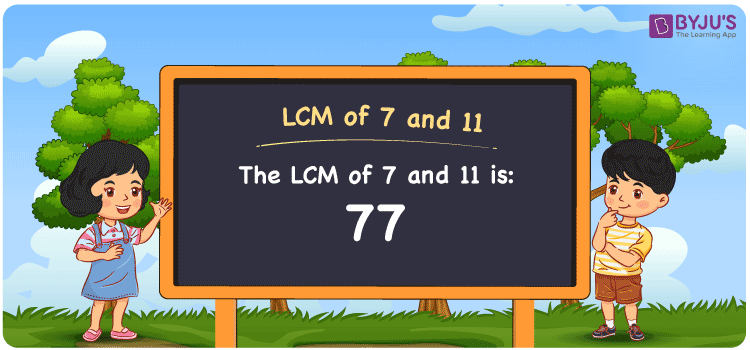Checkout JEE MAINS 2022 Question Paper Analysis : Checkout JEE MAINS 2022 Question Paper Analysis :

# LCM of 7 and 11

LCM of 7 and 11 is 77. The LCM value is the number evenly divisible by the numbers 7 and 11. The multiples which are common among the two numbers will guide you to find the least common multiple of 7 and 11. The multiples of 7 are (7, 14, 21, 28, 35 42, ….) and the multiples of 11 are (11, 22, 33, 44, 55, 66, 77, ….) respectively. Students can find the LCM using methods such as prime factorisation, division and by listing out the multiples of 7 and 11.

## What is LCM of 7 and 11?

The answer to this question is 77. The LCM of 7 and 11 using various methods is shown in this article for your reference. The LCM of two non-zero integers, 7 and 11, is the smallest positive integer 77 which is divisible by both 7 and 11 with no remainder.## How to Find LCM of 7 and 11?

LCM of 7 and 11 can be found using three methods:

• Prime Factorisation
• Division method
• Listing the multiples

### LCM of 7 and 11 Using Prime Factorisation Method

The prime factorisation of 7 and 11, respectively, is given by:

7 = 7¹

11 = 11¹

LCM (7, 11) = 77

### LCM of 7 and 11 Using Division Method

We’ll divide the numbers (7, 11) by their prime factors to get the LCM of 7 and 11 using the division method (preferably common). The LCM of 7 and 11 is calculated by multiplying these divisors.

 7 7 11 11 1 11 x 1 1

No further division can be done.

Hence, LCM (7, 11) = 77

### LCM of 7 and 11 Using Listing the Multiples

To calculate the LCM of 7 and 11 by listing out the common multiples, list the multiples as shown below.

 Multiples of 7 Multiples of 11 7 11 14 22 21 33 28 44 35 55 42 66 49 77 56 88 63 99 70 110 77 121

LCM (7, 11) = 77

## Video Lesson on Applications of LCM## LCM of 7 and 11 Solved Examples

Question: The product of two numbers is 77. Determine the LCM if the GCD is 1.

Solution:

Given

GCD = 1

Product of two numbers = 77

We know that;

LCM x GCD = Product of two numbers

LCM x 1 = 77

LCM = 77/1 = 77

Therefore, the LCM is 77.

## Frequently Asked Questions on LCM of 7 and 11

### Find the GCF if the LCM of 7 and 11 is 77.

We know that

LCM x GCF = 7 x 11

As LCM (7, 11) is 77

77 x GCF = 77

GCF = 77/77 = 1

### What is the LCM of 7 and 11?

The LCM of 7 and 11 is 77. First we should know the multiples of 7 and 11 to find the LCM

Multiples of 7 = 7, 14, 21, 28, 35, ……

Multiples of 11 = 11, 22, 33, 44, 55, 66, ….

The smallest multiple exactly divisible by 7 and 11 is 77.

### Mention the relation between GCF and LCM of 7 and 11.

The relation between GCF and LCM of 7 and 11 is

LCM x GCF = 7 x 11

LCM x GCF = 77